# How to assign values from intervals

The following table presents a list of customers. They are collecting the points.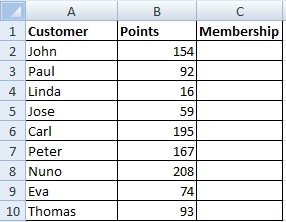Membership depends on the number of points.  The task is to assign correct membership from points intervals.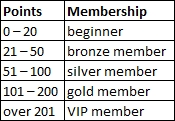## Solution

When you have a multiple conditions to be evaluated, it is not always necessary to use the IF function. In this case, it is best to use the search function VLOOKUP. Let’s see how it works.

### Step 1 / create auxiliary table

The auxiliary table will contains the beginning points of intervals. Intervals have to be sorted in ascending order. This table can be placed anywhere.### Step 2 / use VLOOKUP

VLOOKUP function has 2 variations in finding values. It is to find an exact match and to find the closest match. This behavior affects the last argument Range_Lookup.

Select cell C2 and write this formula:

=VLOOKUP(B2,\$E\$2:\$F\$6,2,1)

or fill VLOOKUP arguments window: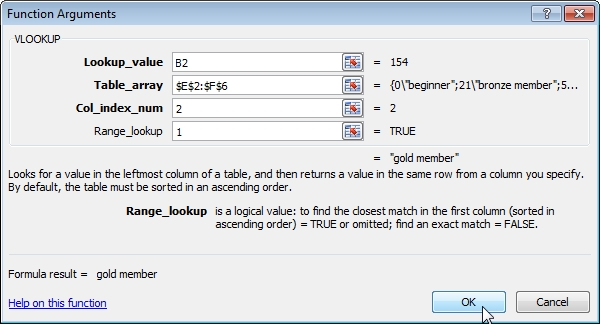Lookup_value is cell B2 (value 154). If this value is not in the first column of Table_array, then will VLOOKUP search for the closest lower value. So, it finds value 101 and result is gold member.

\$ character in the second argument is important, because when you copy this formula, Table_array argument must be still the same. VLOOKUP would not work correctly without the \$ character in the second argument.

Col_index_num is 2, because Membership column is on the second place in the auxiliary table (in the Table_array).

Range_lookup is 1, because we want to find the closest match. Here you can also write the word TRUE.

Now copy formula from C2 to other cells.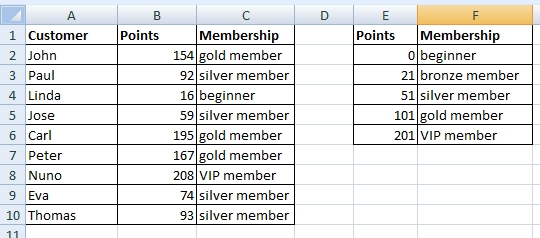1.I’m wondering if there is some overview of all VLOOKUP use cases. This example is pretty cool but I want more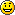2.Best VLOOKUP hint I ever seen.

3.This match value from range is great solution. I am glad that it can be done without macro. Is there any limitation of the source groups? I want to choose values from a big array of conditions. Thank you.

4.Muito bom.
Explicação clara e objetiva.

5.Be careful, this doesn’t work properly!
Ex: with 22 it will return the 21 result (bronze) instead of the 51 (silver), because it searches for the ‘nearest’ value.

BR
CC

•It Do Works

The VLOOKUP starts at the top of the range you specify and looks down (vertically) in each cell to find the value you are looking for (lookup value). It stops searching when it finds a value that is greater than or equal to the lookup value.

6.Excel how to choose value from range. Thank you for this solution.

7.WOW

it’s very simple. I always wonder how is Excel powerful when you are smart and able to use Excel in the proper way.

Btw. Alex Excelnice nickname